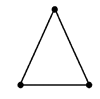NCERT Solutions for Class 6 Maths Chapter 5 Understanding Elementary Shapes Ex 5.6

# NCERT Solutions for Class 6 Maths Chapter 5 Understanding Elementary Shapes Ex 5.6

## NCERT Solutions for Class 6 Maths Chapter 5 Understanding Elementary Shapes Ex 5.6

NCERT Solutions for Class 6 Maths Chapter 5 Understanding Elementary Shapes Ex 5.6 are the part of NCERT Solutions for Class 6 Maths. Here you can find the NCERT Solutions for Class 6 Maths Chapter 5 Understanding Elementary Shapes Ex 5.6.

### Ex 5.6 Class 6 Maths Question 1.

Name the types of following triangles:
(а) Triangle with lengths of sides 7 cm, 8 cm and 9 cm.
(b) ∆ABC with AB = 8.7 cm, AC = 7 cm and BC = 6 cm.
(c) ∆PQR such that PQ = QR = PR = 5 cm.
(d) ∆DEF with m
D = 90°.
(e) ∆XYZ with m
Y = 90° and XY = YZ.
(f) ∆LMN with m
L = 30°, mM = 70° and mN = 80°.

Solution:
(a) The lengths of the three sides of a triangle are given as: 7 cm, 8 cm and 9 cm.
Since, all the sides of the given triangle are different.
Hence, it is a scalene triangle.
(b) Given in a ∆ABC, AB = 8.7 cm, AC = 7 cm and BC = 6 cm.
Here, AB ≠ AC ≠ BC. Hence, ∆ABC is a scalene triangle.
(c) Given in PQR, PQ = QR = PR = 5 cm.
Since all the sides of the triangle are equal.
Hence, it is an equilateral triangle.
(d) Given in ∆DEF, m
D = 90°.
Hence, it is a right-angled triangle.
(e) Given in ∆XYZ, m
Y = 90° and XY = YZ.
Hence, it is an isosceles right-angled triangle.
(f) Given in ∆LMN, m
L = 30°, m M = 70° and mN = 80°.
Hence, it is an acute-angled triangle.

### Ex 5.6 Class 6 Maths Question 2.

Match the following:
Measure of triangle                                       Type of triangle
(i) 3 sides of equal length                                (a) Scalene
(ii) 2 sides of equal length                               (b) Isosceles right angled
(iii) All sides are of different length                (c) Obtuse angled
(iv)  3 acute angles                                          (d) Right angled
(v) 1 right angle                                               (e) Equilateral
(vi) 1 obtuse angle                                             (f) Acute angled
(vii) 1 right angle with two sides of equal length    (g) Isosceles

Solution:
(i) ↔ (e)
(ii) ↔ (g)
(iii) ↔ (a)
(iv) ↔ (f)
(v) ↔ (d)
(vi) ↔ (c)
(vii) ↔ (b)

### Ex 5.6 Class 6 Maths Question 3.

Name each of the following triangles in two different ways: (You may judge the nature of the angle by observation)

Solution:
(a) (i) Acute-angled triangle or (ii) Isosceles triangle

(b) (i) Right-angled triangle or (ii) Scalene triangle

(c) (i) Obtuse-angled triangle or (ii) Isosceles triangle

(d) (i) Right-angled triangle or (ii) Isosceles triangle

(e) (i) Acute-angled triangle or (ii) Equilateral triangle

(f) (i) Obtuse-angled triangle or (ii) Scalene triangle

### Ex 5.6 Class 6 Maths Question 4.

Try to construct triangles using matchsticks. Some are shown here. Can you make a triangle with
(a) 3 matchsticks?
(b) 4 matchsticks?
(c) 5 matchsticks?
(d) 6 matchsticks?

(Remember you have to use all the available matchsticks in each case)
Name the type of triangle in each case.
If you cannot make a triangle, think of reasons for it.

Solution:
(a) Yes, we can make an equilateral triangle with 3 matchsticks.(b) No, we cannot make a triangle with 4 matchsticks.
(c) Yes, we can make an isosceles triangle with 5 matchsticks.(d) Yes, we can make an equilateral triangle with 6 matchsticks.

You can also like these:

NCERT Solutions for Maths Class 7

NCERT Solutions for Maths Class 8

NCERT Solutions for Maths Class 9

NCERT Solutions for Maths Class 10

NCERT Solutions for Maths Class 11

NCERT Solutions for Maths Class 12